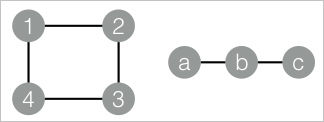In Undirected Graph G, Vertex A is connected to Vertex B if a path exists between the two vertices. Undirected Graph G contains several subgraphs. Each vertex is connected to other vertices in the same subgraph. Vertices in different subgraphs are not connected. In this case, the subgraphs in Undirected Graph G are called maximum connected subgraphs. This topic describes the Maximum Connected Subgraph component provided by Machine Learning Studio.

You can configure the component by using one of the following methods:

## Machine Learning Platform for AI console

Tab Parameter Description
Fields Setting Start Vertex The start vertex column in the edge table.
End Node The end vertex column in the edge table.
Tuning Workers The number of vertices for parallel job execution. The parallelism level and framework communication costs increase with the value of this parameter.
Memory Size per Worker The maximum size of memory that a single job can use. By default, the system allocates 4,096 MB for each job. If the used memory size exceeds the value of this parameter, the OutOfMemory exception is reported.
Data Split Size The data split size. Default value: 64.

## PAI command

``````PAI -name MaximalConnectedComponent
-project algo_public
-DinputEdgeTableName=MaximalConnectedComponent_func_test_edge
-DfromVertexCol=flow_out_id
-DtoVertexCol=flow_in_id
-DoutputTableName=MaximalConnectedComponent_func_test_result;``````
Parameter Required Description Default value
inputEdgeTableName Yes The name of the input edge table. No default value
inputEdgeTablePartitions No The partitions in the input edge table. Full table
fromVertexCol Yes The start vertex column in the input edge table. No default value
toVertexCol Yes The end vertex column in the input edge table. No default value
outputTableName Yes The name of the output table. No default value
outputTablePartitions No The partitions in the output table. No default value
lifecycle No The lifecycle of the output table. No default value
workerNum No The number of vertices for parallel job execution. The parallelism level and framework communication costs increase with the value of this parameter. Not configured
workerMem No The maximum size of memory that a single job can use. By default, the system allocates 4,096 MB for each job. If the used memory size exceeds the value of this parameter, the OutOfMemory exception is reported. 4096
splitSize No The data split size. 64

## Examples

1. Generate training data.
``````drop table if exists MaximalConnectedComponent_func_test_edge;
create table MaximalConnectedComponent_func_test_edge as
select * from
(
select '1' as flow_out_id,'2' as flow_in_id from dual
union all
select '2' as flow_out_id,'3' as flow_in_id from dual
union all
select '3' as flow_out_id,'4' as flow_in_id from dual
union all
select '1' as flow_out_id,'4' as flow_in_id from dual
union all
select 'a' as flow_out_id,'b' as flow_in_id from dual
union all
select 'b' as flow_out_id,'c' as flow_in_id from dual
)tmp;
drop table if exists MaximalConnectedComponent_func_test_result;
create table MaximalConnectedComponent_func_test_result
(
node string,
grp_id string
);``````
The following figure shows the structure of the maximum connected subgraph.2. View training results.
``````+-------+-------+
| node  | grp_id|
+-------+-------+
| 1     | 4     |
| 2     | 4     |
| 3     | 4     |
| 4     | 4     |
| a     | c     |
| b     | c     |
| c     | c     |
+-------+-------+``````